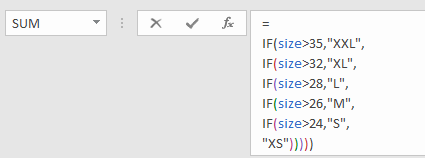Excel allows you to enter a formula with up to 8,192 characters. However, managing a formula will certainly get harder as you get closer to that limit. You might especially have this issue when working with nested formulas - functions in functions. In this guide, we’re going to show you how to make complex formulas easier to read using line breaks in Excel.

In our example, we have table of pants size chart and we are using nested-IF functions get the size code by waist measurement.=IF(size>35,"XXL",IF(size>32,"XL",IF(size>28,"L",IF(size>26,"M",IF(size>24,"S","XS")))))

Obviously, the formula is hard to understand at first glance. There are 5 IF functions with parameters including a named range, numbers, and strings. Let’s see how we can use line breaks to make complex formulas easier to read.

First, increase the formula bar height. Since line breaks mean multiple lines, you might want to adjust the height of the formula bar by holding and dragging the bottom border of the bar.Although each formula structure will contain a different logic, a common nested-IF structure will probably look like this. A new IF function starts with a [value_if_false] argument, thus logical statement and [value_if_true] arguments share the same properties. Based on this assumption, it’s a good practice to group the logical statement and [value_if_true] arguments.

Start by placing your cursor before the first [value_if_false] argument, and press Alt + Enter if you are using Excel for Windows. If you are a Mac user, use⌃ + ⌥ + Return instead.If you are struggling to locate the arguments, you can use Excel’s formula tip popover to ease the process. When you click on a formula, Excel pops over a yellow rectangle that shows the arguments. Click on the argument name to select and highlight the entire argument. You can use this dialog to select the [value_if_false] argument and press the left arrow key (←) to move the cursor before the argument.Continue adding line breaks until the last line. Your formula bar will look similar to this:Although, this is a lot more readable than the original, there is one last step: Add one more line breaks after the equal sign (=) to align all IFs.Far better, right?

=

IF(size>35,"XXL",

IF(size>32,"XL",

IF(size>28,"L",

IF(size>26,"M",

IF(size>24,"S",

"XS")))))